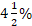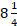• Save

# Simple interest questions and answers for competitive examsThe Quantitative Aptitude section is one of the most difficult chapters in SSC and bank exams, but if it is prepared properly, we can also get better marks. Simple interest is one of those difficult chapters that put the contestants in a lot of trouble and some contestants leave without trying to solve them. Interest is usually paid on an annual, half-yearly, and quarterly basis. As you know, there are two types of interest - simple and compound. When the interest is calculated on the principal for any period of time, it is called simple interest.

Simple interest questions asked in many competitive exams and Simple interest problems are also important for SSC and Bank exams. Here you can practice Simple interest questions with Answers. Here I am sharing 25 important simple interest questions and answers to prepare simple interest quantitive aptitude problems.

## Simple interest questions and answers for SSC bank exams:

1. At the rate ofp.a simple interest, a sum of Rs. 2400 will earn how much interest in 4 years 6 months?

(a) Rs. 796

(b) Rs. 816

(c) Rs. 486

(d) Rs. 956

Ans .   C

2. Ram borrows Rs. 10000 for 2 years at 2% p.a simple interest. He immediately lends it to another person at%  p.a for 2 years. Find his gain in the transaction per year.

(a)  225

(b) 625

(c) 150

(d) 167.50

Ans .   B

3. A sum of Rs. 25000 amounts to Rs. 31000 in 4 years at the rate of simple interest what is the rate of interest?

(a) 3%

(b) 4%

(c) 5 %

(d) 6 %

(e) None of these

Ans .   D

4. Kamla took a loan of Rs. 2400 with simple interest for as many years as the rate of interest. If she paid Rs. 864 as interest at the end of the loan period, what was the rate of interest?

(a) 3.6

(b) 6

(c) 18

(d) cannot determined

(e) None of these

Ans .   B

5. Ram took a loan from a bank at the rate of 24% P.a simple interest. After 6 years he had to pay Rs 10800 interest only for the period. The principal amount borrowed by him was?

(a) 6000

(b) 7500

(c) 7200

(d) 4200

(e) None of these

Ans .   B

6. What is the present worth of Rs. 264 dues in 4 years at 10% simple interest per annum?

(a) 170.20

(b) 166

(c) 188.57

(d) 175.28

Ans .   C

7. A sum fetched a total simple interest of Rs. 8016.25 at the rate of 6 p.c.p.a in 5 years what is the sum?

(a) 24720.83

(b) 26730.33

(c) 26720.83

(d) 26710.63

(e) None of these

Ans .   C

8. Rs. 800 becomes Rs. 956 in 3 years at a certain rate of simple interest. If the rate of interest is increased by 4%, what amount will Rs 800 become in 3 years?

(a) 1020.80

(b) 1025

(c) 1052

(e) None of these

Ans .   C

Ask me in the comment box if you face any problem in solving Simple interest questions, also share this post with friends.